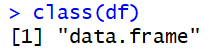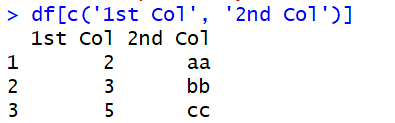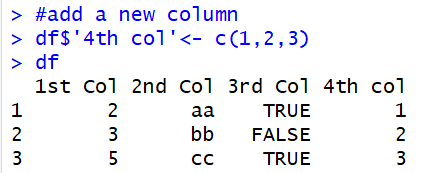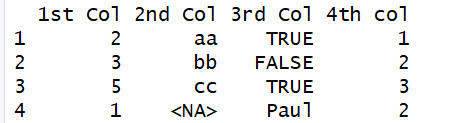# An Easy Guide To Dataframe in R

In this article, we are going to talk about what is dataframe, how to create dataframe in r, access elements of dataframe, update dataframe in r, and delete dataframe.

## What is dataframe?

Dataframe is a way in which we store our data. For example, let’s say you have a dataset in an excel or CSV file and you want to load that file in R. Then you can store that dataset in a dataframe and do all the operation.A dataframe is a list of vectors having the same length. When we say the same/equal length that means the number of elements should be the same in all the vectors. And so, we say a dataframe is also two-dimensional data structure in R.

The other way we can store the dataset are in- Vector, List, Matrix, and dataframe.

The major difference between matrix and dataframe is, the matrix can have only one datatype while dataframe can have a mixture of datatypes. For example, it can have numeric, character, factor, etc.

### Characteristics of dataframe

Here are some of the features of dataframe-

• The column names should be non-empty
• The row names should be unique
• The data stored in a data frame can be of numeric, factor or character type
• Each column should contain the same number of data items

## How to create dataframe in R?

Now as you know what is dataframe, let’s see how to create dataframe in R.

We can create dataframe in R by using the function data.farme()

Syntax:

data.frame(df, stringsAsFactors = FALSE)

Here:

df: It can be a matrix or a dataset that you have just loaded

stringsAsFactors: By default, when we load any dataset in R, R by default consider all string related columns to factors. And so, if we don’t want R to convert string related columns to factor, we can specify stringsAsFactors as FALSE else TRUE. The default behavior is TRUE.

Now let’s see how we can create data frame by list of vectors having same length.

n=c(2,3,5)

s=c(“aa”,”bb”,”cc”)

b=c(TRUE,FALSE,TRUE)

#create a dataframe usig vectors

df=data.frame(n,s,b)

df

Output:So, as we can see all the three vectors have been combined to form the data frame with the name df. Also, each vector will be arranged in the columns. And so, all the three vectors are showing in three columns.

We can see that the column name of the dataframe is the vector name which can be changed. Name() is the function to name the columns of the dataframe. Let’s see how to do it-

names(df) <- c(‘1st Col’, ‘2nd Col’, ‘3rd Col’)

df

Output:Here I have provided the name of the columns as 1st col, 2nd col, and 3rd col respectively.

### Check structure of dataframe

To check the structure of the dataframe, we can use str() function. For example-

str(df)

Output:This output says we have 3 records and 3 columns in the dataframe. Then it shows you the column names followed by the datatype of the columns and then few sample records of each column.

### Check if a variable in dataframe or not?

If we want to check if the given variable or created variable is a dataframe or not, then we can use the class() function.

class(df)

Output:So, this says that “df” is a dataframe.

We can also check if “df” is a dataframe only or not then we can use is.data.frame() function. This results in TRUE/FALSE as output. If the variable will be a dataframe then the output will be true else false.

is.data.frame(df)

Output:

 TRUE

## Dataframe Functions

Here are some of the additional functions which you can use to check the number of rows and columns. Although the same can also be checked by the str() function. But individually you can also check by using ncol() and nrow() functions.

ncol(df)

Output:

3

nrow(df)

Output:

3

## How to access the elements of a dataframe?

Now let’s see how we can access the elements (rows and columns) of a dataframe.

We can access a particular column of a dataframe using the \$ sign. For example, let’s access the 1st col of dataframe df-

df\$`1st Col

Output:

 2 3 5

We can also access more than one column at a time like below-

df[c(‘1st Col’, ‘2nd Col’)]

Output:As we know the data are stored in the form of row:column format. And so, if we want to access the 1st row, we can access like below-

df[1,]

Output:Here we have written df[1,] that means we are looking for 1st row and all other columns. Blank after comma means all columns.

### Add a column to dataframe

We can add a column to an existing dataframe. The condition is the length should be the same and then only we can add a column to the existing dataframe.

For example, let’s add a new column named “4th col” to the existing dataframe df having an element (1,2,3)

df\$’4th col'<- c(1,2,3)

df

Output:

###You can check our detailed guide on add column to dataframe r.

### Add a new row to dataframe

We can also add a new row to the dataframe. To add a new row to the existing dataframe, we can use the function rbind().

rbind(df,list(1,NA,”Paul”,2))

df

Output:

###Delete a column from dataframe

We can also delete a column from a dataframe. For example, let’s delete the first column from the existing dataframe df like below-

df\$`1st Col`<- NULL

df

Output:So, simply, access the column which you want to be deleted and simply assign it to NULL.

### Delete Row from Dataframe

We can also delete a row from a dataframe. For example, let’s delete the 4th row from the dataframe-

df<- df[-4,]

df

Output:So, basically whichever column needs to be deleted, simply put a negative sign behind it and it will be deleted from the dataframe.

We can also delete a column like we deleted the row above. For example-

df<-df[,-2]

df

Output:

##Subset a dataframe

We can create a subset of dataframe from existing dataframe based on some condition.

Syntax:

subset(x, condition)

Arguments:

– x: data frame used to perform the subset

– condition: define the conditional statement

For example, we are looking to select only those records where 4th col value should be more than 2.

subset(df, df\$`4th col`>2)

Output:

##How to update dataframe in R

We can also update the elements of the dataframe in R. To update the elements of the dataframe in R, we just need to select the position of the element and assign the value.

For example, Let’s say we want to update the 1st row, 2nd column record (which is currently 1) to “HDFS” then we can do the following-

df

df[1,2]<- “HDFS”

df

Output:

###Conclusion-

These were all about dataframe in R. In this tutorial, we discussed about the following-

• What is dataframe
• Dataframe features
• How to create a dataframe
• How to update the dataframe
• Adding rows and columns to existing dataframe
• Accessing the elements of the dataframe
• Deleting the rows and columns of the dataframe etc.

Hope you followed the guide on dataframe in R and came this way. Here is the entire code which we have used in this dataframe in R. You can also download the file using the below link.

```n=c(2,3,5)
s=c("aa","bb","cc")
b=c(TRUE,FALSE,TRUE)
#create a dataframe usig vectors #
df=data.frame(n,s,b)
df

#name the columns
names(df) <- c('1st Col', '2nd Col', '3rd Col')
df

#check structure of dataframe
str(df)

#check datatype
class(df)

#check if it is a dataframe
is.data.frame(df)

#check number of columns
ncol(df)

#check number of rows
nrow(df)

#access 1st col
df\$`1st Col`

#access 1st and 2nd column
df[c('1st Col', '2nd Col')]

#access 1st row
df[1,]

df\$'4th col'<- c(1,2,3)
df

#add a row to the dataframe
df<-rbind(df,list(1,NA,"Paul",2))
df

#delete the 1st column
df\$`1st Col`<- NULL
df

#delete 4th row from dataframe
df<- df[-4,]
df

#delete column using -ve sign
df<-df[,-2]
df

# Select only those records where 4th col value should be more than 2
subset(df, df\$`4th col`>2)

#update the element
df
df[1,2]<- "HDFS"
df
```

If you face any issue, please feel free to comment below. You can check more such R Tutorials here.